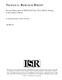## Design Optimization of Multi-Sink Sensor Networks by Analogy to Electrostatic Theory

 dc.contributor.advisor Shayman, Mark en_US dc.contributor.author Kalantari, Mehdi en_US dc.contributor.author Shayman, Mark en_US dc.contributor.department ISR en_US dc.date.accessioned 2007-05-23T10:18:22Z dc.date.available 2007-05-23T10:18:22Z dc.date.issued 2005 en_US dc.description.abstract In this work we introduce a new mathematical tool for optimization of routes, and topology design in wireless sensor networks. We introduce a vector field formulation that models communication in the network, and routing is performed in the direction of this vector field at every location of the network. The magnitude of the vector field at every location represents the density of amount of data that is being transited through that location. We define the total communication cost in the network as the integral of a quadratic form of the vector field over the network area. Our mathematical machinery is based on partial differential equations analogous to the Maxwell equations in electrostatic theory. We use our vector field model to solve the optimization problem for the case in which there are multiple destinations (sinks) in the network. In order to optimally determine the destination for each sensor, we partition the network into areas, each corresponding to one of the destinations. We define a vector field, which is conservative, and hence it can be written as the gradient of a scalar function (also known as a potential function). Then we show that in the optimal assignment of the communication load of the network to the destinations, the value of that potential function should be equal at the locations of all the destinations. Also, we show that such an optimal partitioning of the network load among the destination is unique, and we give iterations to find the optimal solution. en_US dc.format.extent 13854 bytes dc.format.mimetype application/pdf dc.identifier.uri http://hdl.handle.net/1903/6579 dc.language.iso en_US en_US dc.relation.ispartofseries ISR; TR 2005-112 en_US dc.subject Sensor-Actuator Networks en_US dc.title Design Optimization of Multi-Sink Sensor Networks by Analogy to Electrostatic Theory en_US dc.type Technical Report en_US
##### Original bundle
Now showing 1 - 1 of 1